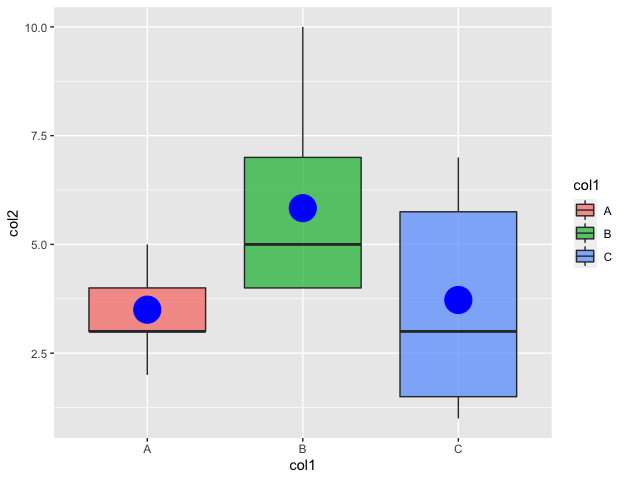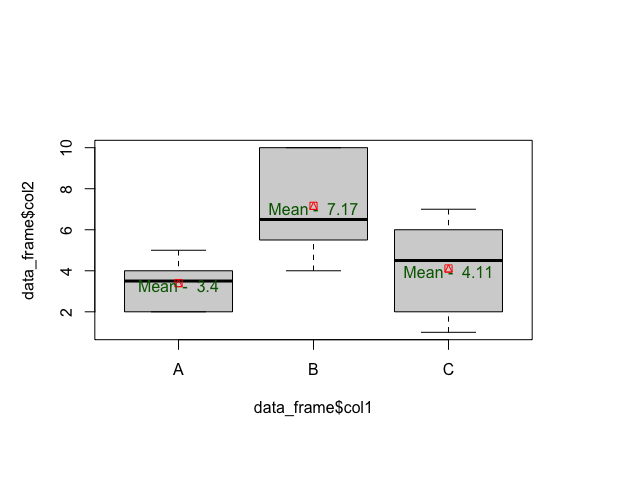Open in App
Not now

# How to plot means inside boxplot using ggplot2 in R?

• Last Updated : 02 Nov, 2022

In this article, we are going to see how to plot means inside boxplot using ggplot in R programming language.

A box plot in base R is used to summarise the distribution of a continuous variable. It can also be used to display the mean of each group. Means or medians can also be computed using a boxplot by labeling points.

## Method 1: Using stat_summary method

The ggplot method in R is used to do graph visualizations using the specified data frame. It is used to instantiate a ggplot object. Aesthetic mappings can be created to the plot object to determine the relationship between the x and y-axis respectively. Additional components can be added to the created ggplot object.

Syntax: ggplot(data = NULL, mapping = aes(), fill = )

Arguments :

• data – Default dataset to use for plot.
• mapping – List of aesthetic mappings to use for plot.

Geoms can be added to the plot using various methods. The geom_boxplot() method in R can be used to add box plots in the plots made. It is added as a component to the existing plot. Aesthetic mappings can also contain color attributes which is assigned differently based on different data frames.

`geom_boxplot(alpha = )`

The method stat_summary() can be used to add mean points to a box plot. It is used to add components to the made plot. This method saves the calculation of mean before plotting the data.

sSyntax: tat_summary(fun=mean, geom=)

Arguments :

• geom – The geometric object to use display the data
• position – The position adjustment to use for overlapping points on this layer

Example:

## R

 `# Library``library``(ggplot2)` `# defining the columns of the data frame``data_frame <- ``data.frame``(col1=``c``(``rep``(``"A"``, 10) ,``                                ``rep``(``"B"``, 12) ,``                                ``rep``(``"C"``, 18)),``                         ``col2=``c``( ``sample``(2:5, 10 ,``                                        ``replace=T) ,``                                ``sample``(4:10, 12 ,``                                       ``replace=T),``                                ``sample``(1:7, 18 ,``                                       ``replace=T))``                         ``)` `# plotting the data frame``graph <- ``ggplot``(data_frame,``                ``aes``(x=col1, y=col2, fill=col1)) +``  ``geom_boxplot``(alpha=0.7) +``  ``stat_summary``(fun=mean, geom=``"point"``,``               ``shape=20, color=``"blue"``,``               ``fill=``"blue"``)` `# constructing the graph``print``(graph)`

Output## Method 2: Using the aggregate method

Aggregate() method in base R is used to split the data into subsets. It can also be used to compute summary statistics for each of the computed subsets and then return the result in a group by form.

Syntax: aggregate(x, by, FUN)

Arguments :

• x – A list or data frame
• by – The list of the column of the data frame to group by
• FUN – The function to apply to x

The boxplot method in R is used to produce box-and-whisker plot(s) of the specified grouped set of values. The boxplot method in R has the following syntax :

Syntax: boxplot( formula)

Arguments :

• formula –  formula, such as y ~ grp, where y is a numeric vector of data values

The boxplot can be customised further to add points and text on the plot.

Syntax: points (x , y , col, pch)

Arguments :

• x ,y – The coordinates of the points to mark
• col – The colour to plot the points with

## R

 `# defining the columns of the data frame``data_frame <- ``data.frame``(col1=``c``(``rep``(``"A"``, 10) ,``                                ``rep``(``"B"``, 12) ,``                                ``rep``(``"C"``, 18)),``                         ``col2=``c``( ``sample``(2:5, 10 ,``                                        ``replace=T) ,``                                ``sample``(4:10, 12 ,``                                       ``replace=T),``                                ``sample``(1:7, 18 ,``                                       ``replace=T))``                         ` `df_col1 <- ``list``(data_frame\$col1)``                         ` `# computing the mean data frame``data_mod <- ``aggregate``(data_frame\$col2,                     ``                        ``df_col1,``                        ``mean)``# plotting the boxplot``boxplot``(data_frame\$col2 ~ data_frame\$col1)``                         ` `# calculating rows of data_mod``row <- ``nrow``(data_mod)``                         ` `# marking the points of the box plot``points``(x = 1:row,                           ``       ``y = data_mod\$x,``       ``col = ``"red"``,``       ``pch = 14``       ``)``                         ` `# adding text to the plot``text``(x = 1:row,  ``     ``y = data_mod\$x - 0.15,``     ``labels = ``paste``(``"Mean - "``, ``round``(data_mod\$x,2)),``     ``col = ``"dark green"``)`

Output:My Personal Notes arrow_drop_up